Related Articles
How to convert categorical data to binary data in Python?
• Last Updated : 13 Jan, 2021

Categorical Data is data that corresponds to the Categorical Variable. A Categorical Variable is a variable that takes fixed, a limited set of possible values. For example Gender, Blood group, a person having country residential or not, etc.

Characteristics of Categorical Data :

• This is mostly used in Statistics.
• Numerical Operation like Addition, Subtraction etc. on this type of Data is not possible.
• All the values of Categorical Data are in Categories.
• It usually uses the Array Data Structure.

Example :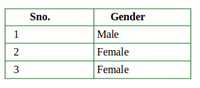Categorical Data

A Binary Data is a Data which uses two possible states or values i.e. 0 and 1.Binary data is mostly used in various fields like in Computer Science we use it as under name Bit(Binary Digit), in Digital Electronic and mathematics we use it as under name Truth Values, and we use name Binary Variable in Statistics.

Characteristics :

• The (0 and 1) also referred to as (true and false), (success and failure), (yes and no) etc.
• Binary Data is a discrete Data and also used in statistics.

Example :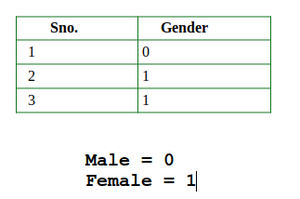Binary Data

## Conversion of Categorical Data into Binary Data

Our task is to convert Categorical data into Binary Data as shown below in python :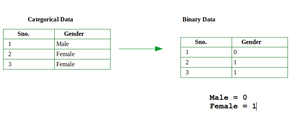Step-by-step Approach:

Step 1) In order to convert Categorical Data into Binary Data we use some function which is available in Pandas Framework. That’s why Pandas framework is imported

## Python3

 `# import required module``import` `pandas as pd`

Step2) After that a list is created and data is entered as shown below.

## Python3

 `# import required modules``import` `pandas as pd`` ` `# assign data``data ``=` `[[``"Jagroop"``, ``"Male"``], [``"Praveen"``, ``"Male"``],``        ``[``"Harjot"``, ``"Female"``], [``"Pooja"``, ``"Female"``],``        ``[``"Mohit"``, ``"Male"``]]`

Step 3) After that Dataframe is created using pd.DataFrame() and here we add extra line i.e. print(data_frame) in order to show the Categorical Data Output as shown below:

## Python3

 `# import required modules``import` `pandas as pd`` ` `# assign data``data ``=` `[[``"Jagroop"``, ``"Male"``], [``"Praveen"``, ``"Male"``],``        ``[``"Harjot"``, ``"Female"``], [``"Pooja"``, ``"Female"``],``        ``[``"Mohit"``, ``"Male"``]]`` ` `# display categorical output``data_frame ``=` `pd.DataFrame(data, columns``=``[``"Name"``, ``"Gender"``])``print``(data_frame)`

Output: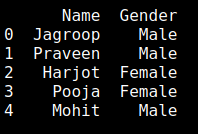Categorical Data

Step 4) Till step 3 we get Categorical Data now we will convert it into Binary Data. So for that, we have to the inbuilt function of Pandas i.e. get_dummies() as shown:

Here we use get_dummies() for only Gender column because here we want to convert Categorical Data to Binary data only for Gender Column.

## Python3

 `# import required modules``import` `pandas as pd`` ` `# assign data``data ``=` `[[``"Jagroop"``, ``"Male"``], [``"Praveen"``, ``"Male"``],``        ``[``"Harjot"``, ``"Female"``], [``"Pooja"``, ``"Female"``],``        ``[``"Mohit"``, ``"Male"``]]`` ` `# display categorical output``data_frame ``=` `pd.DataFrame(data, columns``=``[``"Name"``, ``"Gender"``])``print``(data_frame)`` ` `# converting to binary data``df_one ``=` `pd.get_dummies(data_frame[``"Gender"``])``print``(df_one)`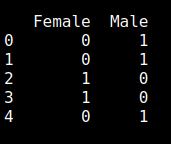output of step 4

Here we get output in binary code for Gender Column only. Here we have two options to use it wisely:

1. Add above output to Dataframe -> Remove Gender Column -> Remove Female column(if we want Male =1 and Female =0) -> Rename Male = Gender -> Show Output of Conversion.
2. Add above output to Dataframe -> Remove Gender Column -> Remove Male column( if we want Male =0 and Female =1) -> Rename Female = Gender -> Show Output of Conversion.

In the below program we used the first option and Write code accordingly as shown below:

## Python3

 `# import required modules``import` `pandas as pd`` ` `# assign data``data ``=` `[[``"Jagroop"``, ``"Male"``], [``"Praveen"``, ``"Male"``],``        ``[``"Harjot"``, ``"Female"``], [``"Pooja"``, ``"Female"``],``        ``[``"Mohit"``, ``"Male"``]]`` ` `# display categorical output``data_frame ``=` `pd.DataFrame(data, columns``=``[``"Name"``, ``"Gender"``])``# print(data_frame)`` ` `# converting to binary data``df_one ``=` `pd.get_dummies(data_frame[``"Gender"``])``# print(df_one)`` ` `# display result``df_two ``=` `pd.concat((df_one, data_frame), axis``=``1``)``df_two ``=` `df_two.drop([``"Gender"``], axis``=``1``)``df_two ``=` `df_two.drop([``"Male"``], axis``=``1``)``result ``=` `df_two.rename(columns``=``{``"Female"``: ``"Gender"``})``print``(result)`

Output: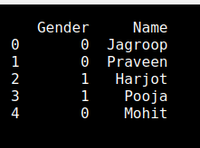Output

Below is the complete program based on the above approach:

## Python3

 `# Pandas is imported in order to use various inbuilt``# Functions available in Pandas framework``import` `pandas as pd`` ` `# Data is initialized here``data ``=` `[[``"Jagroop"``, ``"Male"``], [``"Parveen"``, ``"Male"``],``        ``[``"Harjot"``, ``"Female"``], [``"Pooja"``, ``"Female"``],``        ``[``"Mohit"``, ``"Male"``]]`` ` `# Data frame is created under column name Name and Gender``data_frame ``=` `pd.DataFrame(data, columns``=``[``"Name"``, ``"Gender"``])`` ` `# Data of Gender is converted into Binary Data``df_one ``=` `pd.get_dummies(data_frame[``"Gender"``])`` ` `# Binary Data is Concatenated into Dataframe``df_two ``=` `pd.concat((df_one, data_frame), axis``=``1``)`` ` `# Gendercolumn is droped``df_two ``=` `df_two.drop([``"Gender"``], axis``=``1``)`` ` `# We want Male =0 and Female =1 So we drop Male colunm here``df_two ``=` `df_two.drop([``"Male"``], axis``=``1``)`` ` `# Rename the Column``result ``=` `df_two.rename(columns``=``{``"Female"``: ``"Gender"``})`` ` `# Print the Result``print``(result)`

Output:Output

Attention geek! Strengthen your foundations with the Python Programming Foundation Course and learn the basics.

To begin with, your interview preparations Enhance your Data Structures concepts with the Python DS Course.

My Personal Notes arrow_drop_up